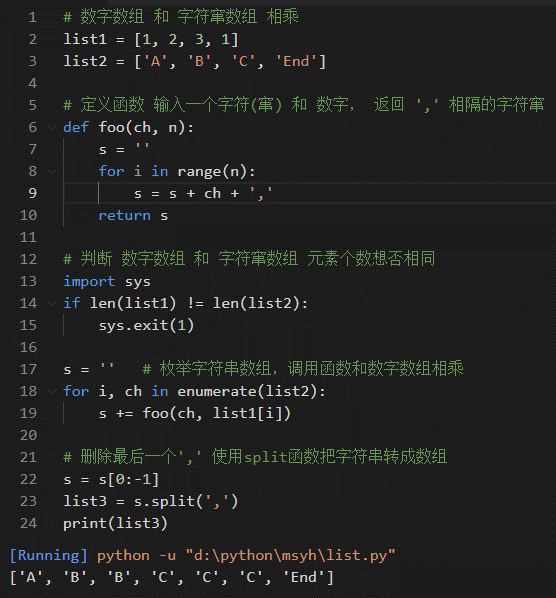﻿ Python和PHP学习: 数字数组 和 字符窜数组 相乘 - 兰雅sRGB个人笔记

C, C++, Python Programming, Source Code, Video

# Python和PHP学习: 数字数组 和 字符窜数组 相乘## 笔记内容: 数组定义操作, if for 复合语句使用， len range enumerate split 函数

### python 版本

``````# 数字数组 和 字符窜数组 相乘
list1 = [1, 2, 3, 1]
list2 = ['A', 'B', 'C', 'End']

# 定义函数 输入一个字符(窜) 和 数字， 返回 ',' 相隔的字符窜
def foo(ch, n):
s = ''
for i in range(n):
s = s + ch + ','
return s

# 判断 数字数组 和 字符窜数组 元素个数想否相同
import sys
if len(list1) != len(list2):
sys.exit(1)

s = ''   # 枚举字符串数组，调用函数和数字数组相乘
for i, ch in enumerate(list2):
s += foo(ch, list1[i])

# 删除最后一个',' 使用split函数把字符串转成数组
s = s[0:-1]
list3 = s.split(',')
print(list3)``````

## PHP版本

``````<?php
# 数字数组 和 字符窜数组 相乘
\$list1 = [1, 2, 3, 1];
\$list2 = ['A', 'B', 'C', 'End'];

# 定义函数 输入一个字符(窜) 和 数字， 返回 ',' 相隔的字符窜
function foo(\$ch, \$n){
\$s = '';
for(\$i = 0; \$i != \$n; \$i++)
\$s .= (\$ch. ',');
return \$s;
}

# 判断 数字数组 和 字符窜数组 元素个数想否相同
if (sizeof(\$list1) != sizeof(\$list2))
exit;

\$s = '';   # 枚举字符串数组，调用函数和数字数组相乘
for (\$i = 0; \$i != sizeof(\$list2); \$i++)
\$s .= foo(\$list2[\$i], \$list1[\$i]);

# 删除最后一个',' 使用split函数把字符串转成数组
\$s = rtrim(\$s, ',');    # rtrim — 删除字符串末端的空白字符（或者其他字符）
\$list3 = explode(',', \$s);  # explode — 使用一个字符串分割另一个字符串
print_r(\$list3);        # var_dump(\$list3);
``````